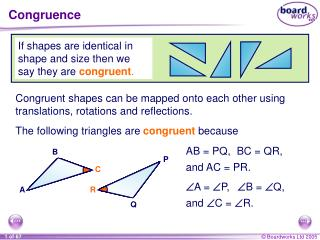Download PresentationCongruence# Congruence - PowerPoint PPT Presentation

Download Presentation##### Congruence

Download Policy: Content on the Website is provided to you AS IS for your information and personal use and may not be sold / licensed / shared on other websites without getting consent from its author. While downloading, if for some reason you are not able to download a presentation, the publisher may have deleted the file from their server.

- - - - - - - - - - - - - - - - - - - - - - - - - - - E N D - - - - - - - - - - - - - - - - - - - - - - - - - - -
##### Presentation Transcript

1. Congruence B B B B B B B P P P P P P P C C C C C C C A A A A A A A R R R R R R R Q Q Q Q Q Q Q If shapes are identical in shape and size then we say they arecongruent. Congruent shapes can be mapped onto each other using translations, rotations and reflections. The following triangles are congruent because AB = PQ, BC = QR, and AC = PR. A = P, B = Q, and C = R.

2. Congruent triangles 1) The three sides of one triangle are equal to the three sides of the other. Side, side side (SSS) Two triangles are congruent if the satisfy the following conditions:

3. Congruent triangles 2) Two sides and the included angle in one triangle are equal to two sides and the included angle in the other. Side, angle, side (SAS) Two triangles are congruent if the satisfy the following conditions:

4. Congruent triangles 3) Two angles and one side of one triangle are equal to the corresponding two angles and side in the other. Angle, angle, side (AAS) Two triangles are congruent if the satisfy the following conditions:

5. Congruent triangles Two triangles are congruent if the satisfy the following conditions: 4) The hypotenuse and one side of one right-angled triangle is equal to the hypotenuse and one side of another right-angled triangle. Right angle, hypotenuse, side (RHS)

6. Congruent triangles

7. S1 Lines, angles and polygons S1.1 Parallel lines and angles • A Contents S1.2 Triangles • A S1.3 Quadrilaterals • A S1.6 Similarity S1.4 Angles in polygons • A S1.5 Congruence • A • A

8. Similar shapes Q B Q Q C B R B Q B C A R C R Q B Q C A R B P A C R A C P R P A A P P P Q B C R A QR PQ PR P = = AB AC BC If one shape is an enlargement of the other then we say the shapes are similar. The angles in two similar shapes are the same size and the lengths of their corresponding sides are in the same ratio. A similar shape can be a reflection or a rotation of the original. The following triangles are similar because A =  P, B = Q, and C = R.

9. Similar shapes Which of the following shapes are always similar? Any two rectangles? Any two squares? Any two isosceles triangles? Any two circles? Any two equilateral triangles? Any two cubes? Any two cylinders?

10. Finding the scale factor of an enlargement length on enlargement Scale factor = corresponding length on original We can find the scale factor for an enlargement by finding the ratio between any two corresponding lengths. If a shape and its enlargement are drawn to scale, the the two corresponding lengths can be found using a ruler. Always make sure that the two lengths are written using the same units before dividing them.

11. Finding the scale factor of an enlargement The following rectangles are similar. What is the scale factor for the enlargement? 6 cm 9 cm The scale factor for the enlargement is 9/6 = 1.5

12. Finding the lengths of missing sides The following shapes are similar. What is the size of each missing side and angle? 3.6 cm c 3 cm 37° 6 cm a 37° 4.8 cm 5 cm e 4 cm 53° b 53° 6 cm 7.2 cm d The scale factor for the enlargement is 6/5 = 1.2

13. Finding lengths in two similar shapes

14. Similar triangles 1

15. Similar triangles 2

16. Using shadows to measure height In ancient times, surveyors measured the height of tall objects by using a stick and comparing the length of its shadow to the length of the shadow of the tall object.

17. Using shadows to measure height